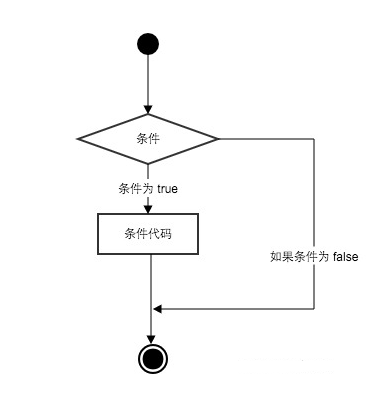# Python if条件控制

Python条件语句是通过一条或多条语句的执行结果（True或者False）来决定执行的代码块。## if 语句

Python中if语句的一般形式如下所示：

``````if condition_1:
statement_block_1
elif condition_2:
statement_block_2
else:
statement_block_3``````

• 如果 “condition_1” 为 True 将执行 “statement_block_1” 块语句
• 如果 “condition_1” 为False，将判断 “condition_2”
• 如果”condition_2″ 为 True 将执行 “statement_block_2” 块语句
• 如果 “condition_2” 为False，将执行”statement_block_3″块语句

Python 中用 elif 代替了 else if，所以if语句的关键字为：if – elif – else

• 1、每个条件后面要使用冒号 :，表示接下来是满足条件后要执行的语句块。
• 2、使用缩进来划分语句块，相同缩进数的语句在一起组成一个语句块。
• 3、在Python中没有switch – case语句。

##### 实例

``````#!/usr/bin/python3

var1 = 100
if var1:
print ("1 - if 表达式条件为 true")
print (var1)

var2 = 0
if var2:
print ("2 - if 表达式条件为 true")
print (var2)
print ("Good bye!")``````

`1 - if 表达式条件为 true100Good bye!`

##### 实例

``````#!/usr/bin/python3

age = int(input("请输入你家狗狗的年龄: "))
print("")
if age < 0:
print("你是在逗我吧!")
elif age == 1:
print("相当于 14 岁的人。")
elif age == 2:
print("相当于 22 岁的人。")
elif age > 2:
human = 22 + (age -2)*5
print("对应人类年龄: ", human)

### 退出提示
input("点击 enter 键退出")``````

`\$ python3 dog.py 请输入你家狗狗的年龄: 1相当于 14 岁的人。点击 enter 键退出`

`<` 小于
`<=` 小于或等于
`>` 大于
`>=` 大于或等于
`==` 等于，比较对象是否相等
`!=` 不等于

##### 实例

``````#!/usr/bin/python3

# 程序演示了 == 操作符
# 使用数字
print(5 == 6)
# 使用变量
x = 5
y = 8
print(x == y)``````

`FalseFalse`

high_low.py文件演示了数字的比较运算：

##### 实例

``````#!/usr/bin/python3

# 该实例演示了数字猜谜游戏
number = 7
guess = -1
print("数字猜谜游戏!")
while guess != number:
guess = int(input("请输入你猜的数字："))

if guess == number:
print("恭喜，你猜对了！")
elif guess < number:
print("猜的数字小了...")
elif guess > number:
print("猜的数字大了...")``````

`\$ python3 high_low.py 数字猜谜游戏!请输入你猜的数字：1猜的数字小了...请输入你猜的数字：9猜的数字大了...请输入你猜的数字：7恭喜，你猜对了！`

## if 嵌套

``````if 表达式1:
语句
if 表达式2:
语句
elif 表达式3:
语句
else:
语句
elif 表达式4:
语句
else:
语句``````

##### 实例

``````# !/usr/bin/python3

num=int(input("输入一个数字："))
if num%2==0:
if num%3==0:
print ("你输入的数字可以整除 2 和 3")
else:
print ("你输入的数字可以整除 2，但不能整除 3")
else:
if num%3==0:
print ("你输入的数字可以整除 3，但不能整除 2")
else:
print  ("你输入的数字不能整除 2 和 3")``````

`\$ python3 test.py 输入一个数字：6你输入的数字可以整除 2 和 3`